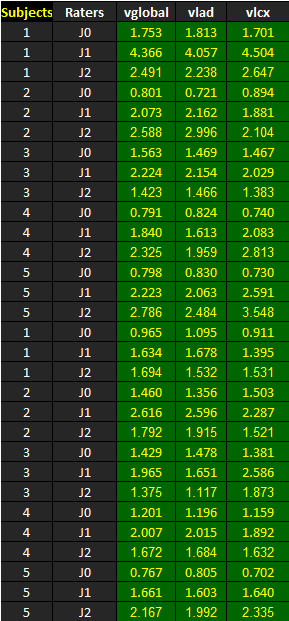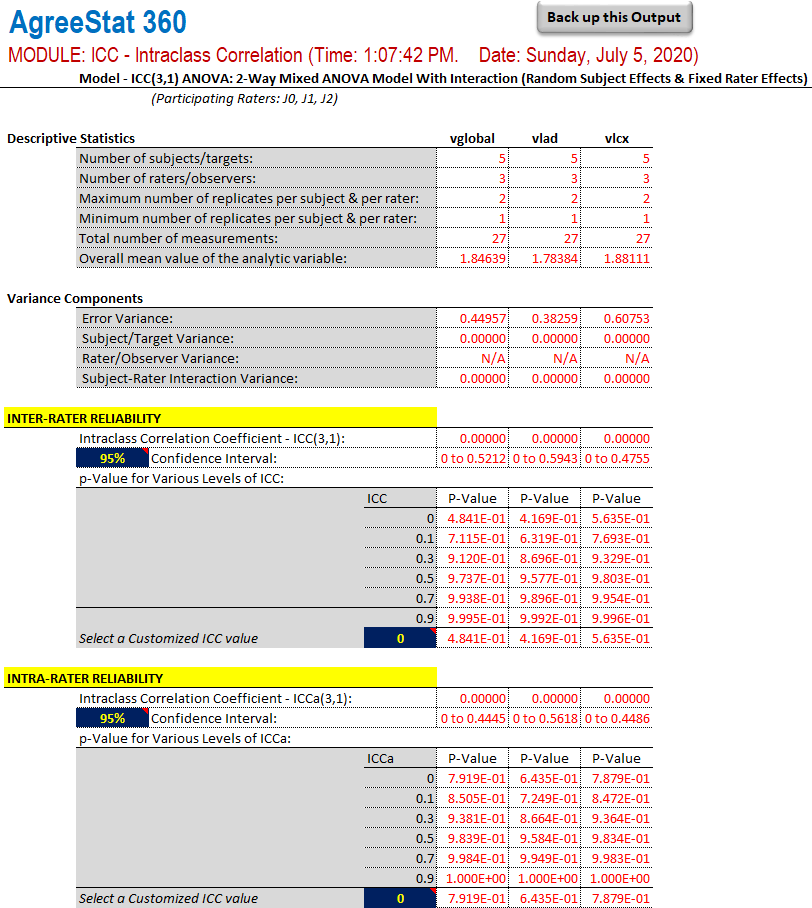﻿ AgreeStat/360: intraclass correlation coefficients for multiple raters under the two-way mixed effects model, for multiple variablesAgreeStat Analytics

## Intraclass Correlation with Multiple Variables 2-Way Mixed Effects Model

Input Data

The dataset shown below is included in one of the example worksheets of AgreeStat 360 (This video shows how you may import your own data to AgreeStat360). It contains the ratings that 3 judges J0, J1, and J2 assigned to 5 subjects.  Note that the 5 subjects were rated on 3 different variables vglobal, vlad, and vlcx.

The goal is to compute the Intraclass Correlation Coefficients (ICC)  under the 2-way mixed effects model, where the rater effect is fixed, and the subject effect random. The analysis must be run independently for each variable.Analysis with AgreeStat/360

To see how AgreeStat360 processes this dataset to produce various agreement coefficients, please play the video below.  This video can also be watched on youtube.com for more clarity if needed.

Results

The output that AgreeStat360 produces is shown below and has 4 parts. The basic descriptive statistics constitute the first part.  The second part shows different variance components, while the third and fourth parts shows the inter-rater and intra-rater reliability estimates respectively, along with their precision measures.

Each of the 4 parts of this output contains statistics separately for each of the 3 analytic variables vglobal, vlad, and vlcx.

• Descriptive statistics: The first part of this output displays basic statistics about the number of non-missing observations for each variable as well as their mean values or number subjects.

• Variance components: This section will typically show the error variance, the subject variance, the rater variance, as well as the subject-rater interaction variance. These variance components are used for calculating the inter-rater and intra-rater reliability coefficients, and could help explain the magnitude of the reliability coefficients.

• Inter-rater reliability: This part shows the inter-rater reliability coefficient for each of the 3 analytic variables, their confidence intervals and associated p-values.  You can modify both the confidence level and the ICC null value to obtain the corresponding confidence intervals and p-values.  The blue cells contain dropdown lists contains new confidence levels and ICC null values to choose from.

• Intra-rater reliability
This part shows the intra-rater reliability coefficient for each of the 3 analytic variables, their confidence intervals and associated p-values.  Again, you can modify both the confidence level and the ICC null value to update the corresponding confidence intervals and p-values.